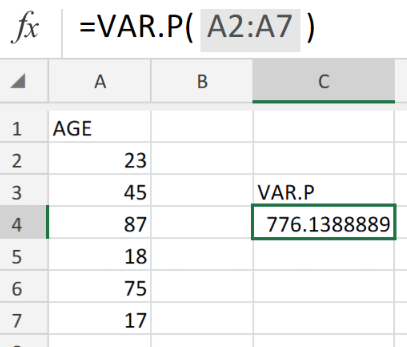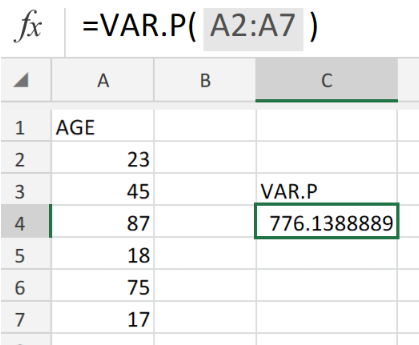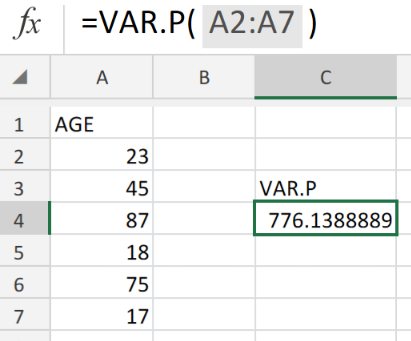Get instant live expert help with Excel or Google Sheets“My Excelchat expert helped me in less than 20 minutes, saving me what would have been 5 hours of work!”

#### Post your problem and you’ll get Expert help in seconds.

Your message must be at least 40 characters
Our professional Expert are available now. Your privacy is guaranteed.

# Excel VAR.P FunctionFigure 1.VAR.P Function in Excel

Let’s assume that we have a range of data representing a population sample, we are going to make use of the Excel VAR.P function to determine the of variance the entire population.

## Generic Formula

`=VAR.P (number1, [number2], ...)`

• number1 is the first reference or number,
• number2 is the second reference or number (optional).

Variance is a measure of how far a set of data is spread out, providing us with a general idea of our data spread.

## How to use the Excel VAR.P Function.

We are now going to demonstrate the use of the Excel VAR.P Function in calculating the variance based on the entire population.

We can achieve this by following 2 simple steps;

1. List the sample data of the population in a column of our worksheet.

See illustration below;Figure 2. Sample Data in Excel

Our purpose here is to determine the variance of a range of ages in a sample population.

Be sure to leave a blank cell for Excel to return the variance value. Cell C4 of our worksheet example.

1. The VAR.P formula which we will enter into cell C4 is;.

`=VAR.P(A2:A7)`Figure 3. Excel VAR.P Function

The cell range A2:A7 represent the individual ages of our sample population.

## Note

• Even though our data set represents a sample of the population, the Excel VAR.P Function will assume that it represents the entire population.Figure 3. Final Result

## Instant Connection to an Expert through our Excelchat Service:

Our live Excelchat Service is here for you. We have Excel Experts available 24/7 to answer any Excel questions you may have. Guaranteed connection within 30 seconds and a customized solution for you within 20 minutes